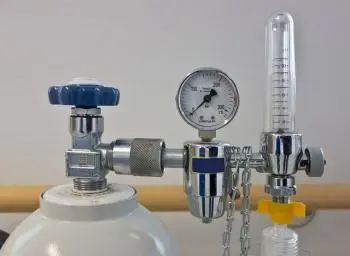# Boyle-mariotte Law: Formula, Examples, Solved ExercisesThe Boyle-Mariotte Law (or simply Boyle's Law) is a law that establishes the relationship between pressure and volume in the thermodynamic processes of a gas.

Boyle's Law is one of the fundamental laws of gas physics and is widely applied in areas such as thermodynamics, physics, chemistry, and engineering. It is especially useful in the study of the behavior of gases and has practical applications in gas compression, ventilation systems, engine design, refrigeration systems, and many other gas-related fields.

The Boyle-Mariotte law was first formulated in the 17th century by the English scientist Robert Boyle, who performed a series of experiments to investigate the properties of gases. However, it was rediscovered soon after by the French scientist Edme Mariotte.

## What Does Boyle's Law State?

Boyle's Law states that, at constant temperature, the volume of a fixed amount of gas is inversely proportional to the pressure applied to it.

In other words, when the temperature is held constant, if the pressure of a gas increases, its volume decreases, and if the pressure decreases, the volume increases.

This law is based on the idea that, at the microscopic level, gases consist of constantly moving particles. When the pressure on a gas is increased, the particles are compressed more tightly, reducing the space they occupy and decreasing the total volume of the gas.

Conversely, if the pressure is reduced, the particles have more space to move and the gas expands, occupying a larger volume.

## Boyle-mariotte Law Formula

Mathematically, Boyle's Law is expressed by the formula:

P₁ * V₁ = P₂ * V₂

Where:

• P₁ and V₁ represent the initial pressure and volume of the gas.

• P₂ and V₂ represent the final pressure and volume of the gas.

## Examples of Boyle's Law

Here are some examples of Boyle's Law that illustrate the relationship between pressure and volume of a gas at constant temperature:

1. Inflatable balloon: If you have an inflatable balloon and you compress it by applying pressure to it, its volume will decrease. As the pressure increases, the gas inside the balloon is compressed and takes up less space, causing the balloon to shrink. On the contrary, by releasing the pressure, the volume of the gas increases and the balloon inflates again.

2. Syringe: Pulling back on the plunger of a medical syringe reduces the volume inside the syringe. This creates a lower pressure in the syringe space, making it possible to draw in liquids or medications. Pushing the plunger forward increases the pressure and expels fluid from the syringe.

3. Car tires: Car tires contain compressed air. If you need to increase tire pressure, you use an air pump to add more air to the inside of the tire. As the pressure increases, the air volume decreases and the tire inflates. On the other hand, if you decrease the pressure, the tire goes flat and the air volume increases.

4. Diving: When a diver descends to greater depths under water, the pressure increases considerably. This causes a decrease in the volume of air in the lungs. If the diver does not gradually exhale the compressed air upon ascent, he or she may experience pulmonary barotrauma due to the sudden expansion of air in the lungs as the pressure decreases.

## Importance of Boyle's Law

The Boyle-Mariotte law is especially useful in fields such as thermodynamics, physics, and chemistry. For example, in industry, this law is applied to the compression of gases for storage and transportation.

In addition, it is essential in the design and operation of internal combustion engines, refrigeration and air conditioning systems, and in the manufacture of chemical products.

Another important application of this law is found in medicine. For example, in the field of pulmonary ventilation, the Boyle-Mariotte law is essential to understanding how the lungs expand and contract during respiration. The increase in pressure in the lungs during inspiration causes a decrease in their volume, allowing air to enter.

## Solved Exercises on the Boyle-mariotte Law

### Exercise 1

A gas occupies a volume of 4 liters at a pressure of 3 atmospheres. If the pressure is reduced to 2 atmospheres, what will be the new volume of the gas?

Solution:

Using Boyle's Law formula (P₁ * V₁ = P₂ * V₂), we can solve the problem.

• P₁ = 3 atm
• V₁ = 4L
• P₂ = 2 atm
• V₂ = ?

Applying the formula:

P₁ * V₁ = P₂ * V₂

(3 atm) * (4 L) = (2 atm) * V₂

12 L atm = 2 atm * V₂

Dividing both sides of the equation by 2 atm:

(12 L atm) / (2 atm) = V₂

V₂ = 6L

Therefore, the new volume of the gas will be 6 liters when the pressure is reduced to 2 atmospheres.

### Exercise 2

A cylinder contains a gas at a pressure of 2 atmospheres and a volume of 10 liters. If the pressure is increased to 4 atmospheres, what will be the new volume of the gas?

Solution:

Using Boyle's Law formula (P₁ * V₁ = P₂ * V₂), we can solve the problem.

• P₁ = 2 atm
• V₁ = 10L
• P₂ = 4 atm
• V₂ = ?

Applying the formula:

P₁ * V₁ = P₂ * V₂

(2 atm) * (10 L) = (4 atm) * V₂

20 L atm = 4 atm * V₂

Dividing both sides of the equation by 4 atm:

(20 L atm) / (4 atm) = V₂

V₂ = 5L

Therefore, the new volume of the gas will be 5 liters when the pressure increases to 4 atmospheres.

Author:

Published: June 5, 2023
Last review: June 5, 2023